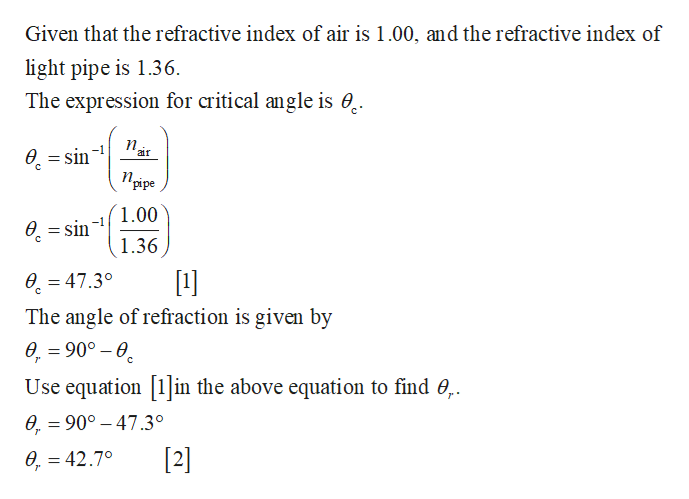Question
16 views

Determine the maximum angle u
for which the light rays incident on
the end of the light pipe in Figure
P22.38 are subject to total internal
reflection along the walls of the
pipe. Assume the light pipe has an
index of refraction of 1.36 and the
outside medium is air.

check_circle

Step 1help_outlineImage TranscriptioncloseGiven that the refractive index of air is 1.00, and the refractive index of light pipe is 1.36. The expression for critical angle is 0. п, 0. = sin air Пруре gipe 1.00 0. = sin 1.36  0. = 47.3° The angle of refraction is given by 0. = 90° – 0. Use equation in the above equation to find 0,. 0, = 90° – 47.3°  0, = 42.7° %3D fullscreen

### Want to see the full answer?

See Solution

#### Want to see this answer and more?

Solutions are written by subject experts who are available 24/7. Questions are typically answered within 1 hour.*

See Solution
*Response times may vary by subject and question.
Tagged in

### Physics